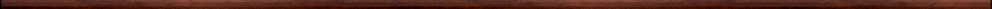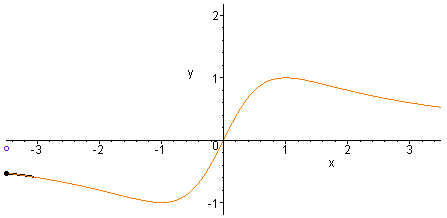Maple worksheets on derivativesBasic calculus topics:

They are all compatible with Classic Worksheet Maple 10.Derivatives from first principles - deriv1.mws

• The derivatives or gradient function associated with a function f(x).
• The standard limit formula for the derivative of a function.
• Examples of determining derivatives from first principles.
• Examples of derivatives of functions of the form f(x)=x^r.
• The formula for the derivatives of a function of the form f(x)=x^r.
• More examples of determining derivatives of functions from first principles.

A procedure which performs differentiation from first principles - deriv2.mws

• A procedure for showing the steps of differentiation from1st principles: diffbylimit.
• Examples of the form: d/dx [x^r].
• Examples of the form: d/dx [q(x)] where q(x) is a polynomial or rational function of x.
• Examples of the form: d/dx [q(x)^r] where q(x) is a polynomial or rational function of x.

Graphs of derivatives - drvgrph.mws

• Graphs of derivatives.
• An animation procedure for graphs of derivatives: derivplot.
• Tangents which meet a curve at another point.

The power rule, linearity of differentiation and Leibniz notation - rules1.mws

• The power rule for differentiation.
• The sum rule for differentiation.
• The restricted rule for multiplication by a constant.
• Leibniz notation.
• Setting up a Maple procedure to perform differentiation.
• The Maple procedure: diff
• The differential operator D.

More differentiation rules - rules2.mws

• The chain rule for differentiation.
• The product rule for differentiation.
• The quotient rule for differentiation.

Tangent and normal lines to curves - tangents.mws

• The point-slope equation of a line.
• Perpendicular lines and the normal line to a curve at a point.
• Examples of finding equations of tangent and normal lines to curves.
• Tangents which meet a curve at another point.

The meaning and uses of the derivative of a function - changes.mws

• The derivative and small changes.
• Average and instantaneous rates of change.
• An example to introduce the notions of differentiability and non-differentiability of a function.

Geometrical interpretation of derivatives - statpts.mws

• Stationary points.
• Comparison of graphs of function and derivative.

Calculus procedures - calculus.zip

Top of page

Main index